# Use Decision Trees in Machine Learning to Predict Stock Movements

Machine Learning for trading is the new buzz word today and some of the tech companies are doing wonderful unimaginable things with it. Today, we’re going to show you, how you can predict stock movements (that’s either up or down) with the help of ‘Decision Trees’, one of the most commonly used ML algorithms.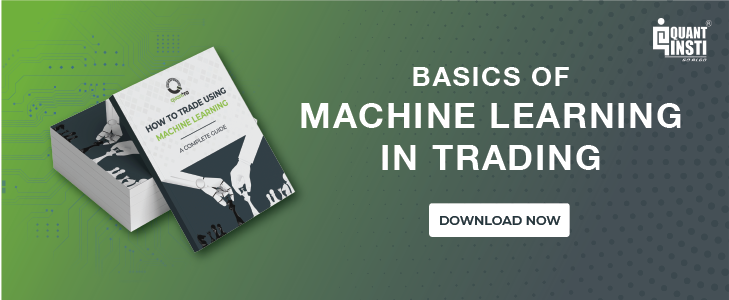Decision trees in Machine Learning are used for building classification and regression models to be used in data mining and trading. A decision tree algorithm performs a set of recursive actions before it arrives at the end result and when you plot these actions on a screen, the visual looks like a big tree, hence the name ‘Decision Tree’.

Here’s an example of a simple decision tree in Machine Learning.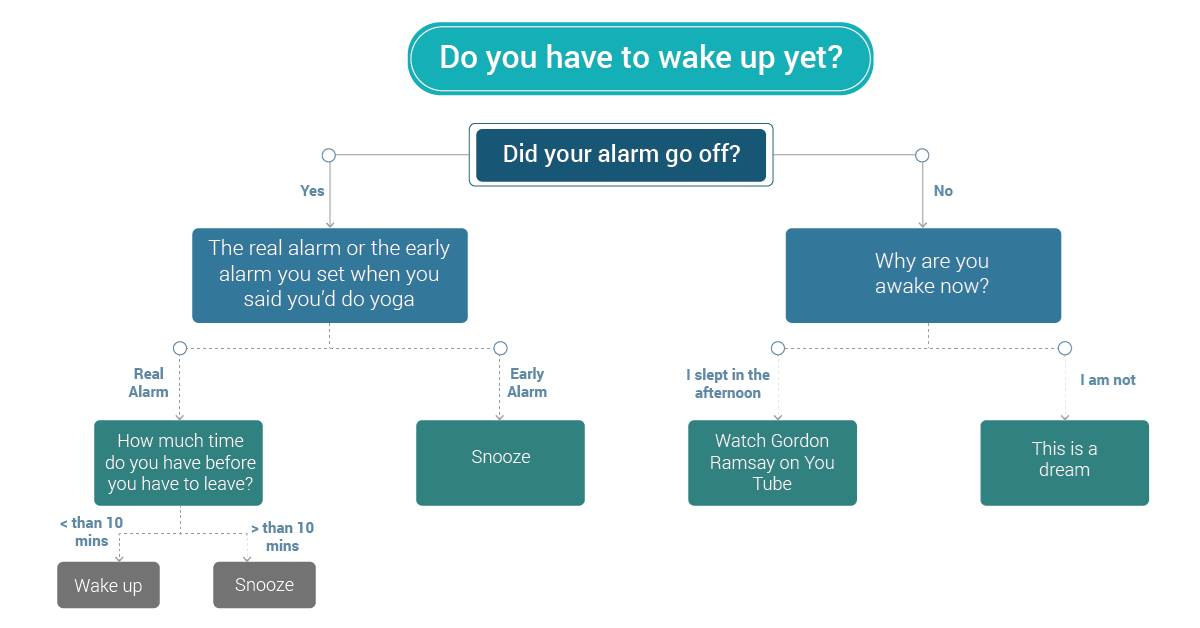Basically, a decision tree is a flowchart to help you make decisions. Machine Learning uses the same technique to make better decisions, let’s find out how.

### Visualizing a sample dataset and decision tree structure

Now let’s come to the point, we want to predict which way your stock will go using decision trees in Machine Learning. We’ll need past data of the stock for that. Consider a sample stock dataset as shown in the table below. The dataset comprises of Open, High, Low, Close Prices and Volume indicators (OHLCV). You can download historical data for any stock using Yahoo finance or Google finance.

We will be doing this exercise in R programming language, you’ll have to install the supporting software on your mac/pc. Here is a link to help you with that. And here’s the code for importing this data onto (The data files including the code will be available at the end of the blog)-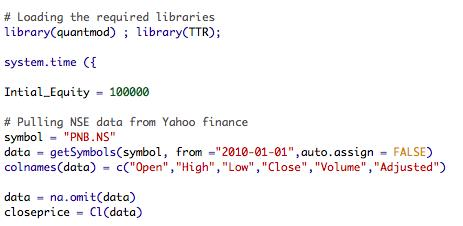And here’s the visual representation of the data.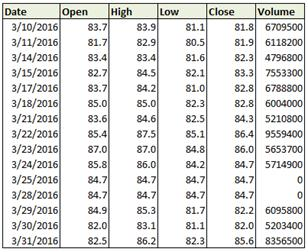Let us add some technical indicators (RSI, SMA, LMA, ADX) to this dataset. Technical indicators are calculated using basic stock values (OHLC) in our case and they help us predict stock movements. Our machine learning algorithm will make use of the values from the technical indicators to make more accurate stock price prediction. We lag the technical indicator values to avoid look-ahead bias.

We also add the “Class” column which signifies the daily returns based on the close price of the stock. In the “Class” column, “Up” denotes positive return, whereas “Down” signifies a negative return. Here’s the code for the same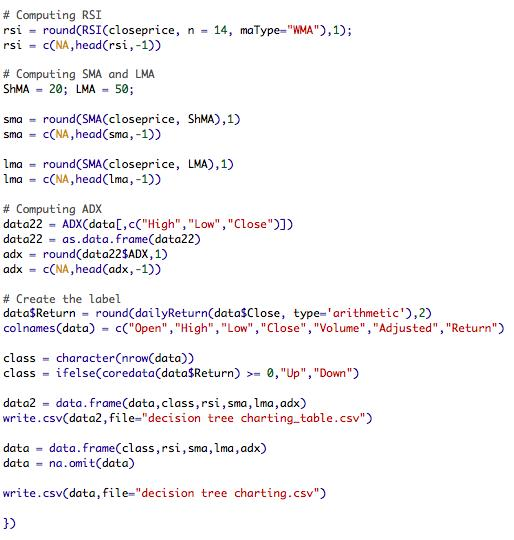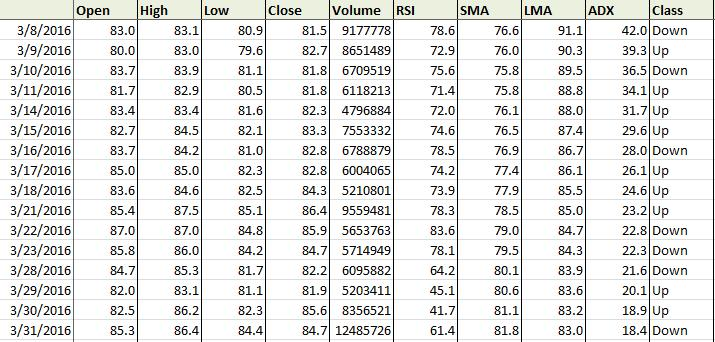Now comes the fun part, we want to predict the daily change (Up/Down) using these technical indicators. We will do this by applying a machine learning decision tree algorithm on this dataset. First, we will have to split our dataset into two parts; training dataset and test dataset. The algorithm uses the training data to learn about the stock’s movements and it makes certain assumptions, this is also called as ‘information gain’. And once the training is done we apply it on a test dataset to make the stock price prediction. This type of Machine Learning is called as supervised learning. Here’s the code for applying these steps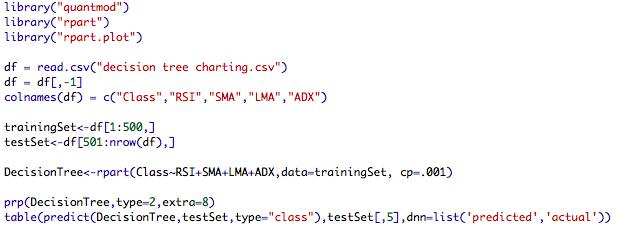The resulting output from the training dataset can be viewed in the form of a tree structure as shown below.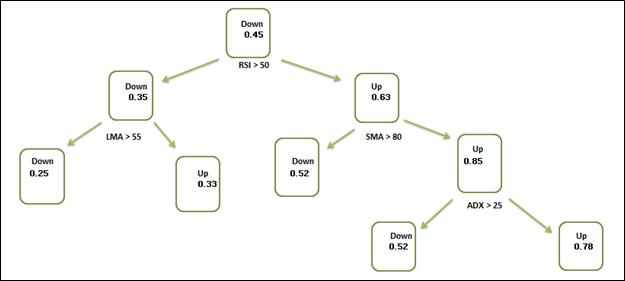Sample illustration of a Decision Tree

Before we understand how the decision tree algorithm of Machine Learning works, let us first understand the tree structure.

### Components of a decision tree

A decision tree structure consists of a root node, test nodes, and decision nodes (leaves). The root node is the main node in a decision tree. In the above decision tree, we start with RSI>50, thus the RSI indicator is used as the root node. You might ask why RSI indicator’s value is at 50 and why not say 34? Here’s where the training comes into the picture, the Machine Learning decision tree algorithm was able to understand that RSI at 50 helps in making more accurate decisions than at any other value.

After the root node, each test node splits the data into further parts according to some set criteria. In the decision tree above, we have SMA> 80, LMA>55, and ADX>25 (again these values are the result of the training). The data is split further based on these indicators which act as test nodes.

The final node is the leaf node. In the decision tree diagram, the nodes containing the Up/Down class along with the probability value indicating the probability of the target attribute are the leaf nodes. The left of any node is considered as ‘yes’, meaning the question asked at the node (e.g. RSI >50 if answered yes, the algorithm navigates to left side of the tree and if not then right)

Thus, the entire dataset gets classified by navigating from the root node of the decision tree down to the leaf, according to the set criteria.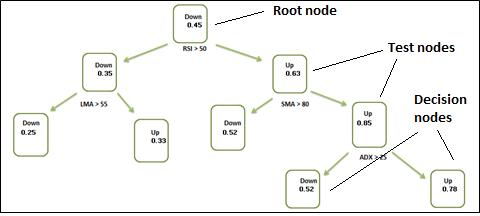Components of a Decision Tree

So for example, this stock on a given day had its RSI at 39 then, so travel to the right of the tree check if the SMA is > 80, if yes then travel to the leaf which says, ‘the stock has a 0.52 probability of going down’.

### How does a decision tree algorithm work?

Now that we have understood the decision tree structure, let us understand how a decision tree is constructed.

##### Decision Tree Inducer

A decision tree is constructed by a decision tree inducer (also called as classifier). There are various decision tree inducers such as ID3, C4.5, CART, CHAID, QUEST, CRUISE, etc. etc. (you can find more information on these inducers here and here) A decision tree inducer is basically an algorithm that automatically constructs a decision tree from a given (training) dataset. Typically, the goal of a decision tree inducer is to construct an optimal decision tree based on a specified target function. An example of a target function can be a minimization of the number of nodes of the decision tree, so as to reduce the complexity. Another example can be minimizing the generalization error (or get more accurate results).

Although we said that the inducer constructs a decision tree automatically, in reality, the decision tree inducer follows a certain method. There are two prevalent methods used by the decision tree inducers in general. These include the widely used top-down method and the less popular, bottom-up method.

##### Top-down Method Explained

Under the top-down method, an inducer creates the decision tree in a top-down, recursive manner. Let us use our previously created technical indicators to understand this top-down, recursive method.

The technical indicators that we created were RSI, SMA, LMA, and the ADX indicator. These indicators are considered as the attributes by our decision tree algorithm.

From among these indicators (attributes), which of the indicator (attribute) will be used by the inducer as the root node to start with? How will the remaining attributes be split?

All this is decided by the inducer using a discrete splitting function. The discrete splitting function uses certain criteria (e.g. information gain, gini index,) to determine the best attribute to start with and split the training dataset. Here are some links to understand more about Information gain, Gini index.

In each iteration, the inducer algorithm partitions the training dataset using the outcome of a discrete function of the input attribute. After the selection of an appropriate split, each node further subdivides the training set into smaller subsets, until no further splitting is possible or when a stopping criterion is fulfilled. After the complete creation of the tree, it is pruned using certain pruning rules to reduce classiﬁcation errors.

This is how a decision tree gets constructed which can be used for making stock price prediction in machine learning. In our future posts, we will demonstrate how to construct a decision tree in python and will also explore some machine learning models based on decision trees.

### Next Step

Sign up for our latest course on ‘Decision Trees in Trading‘ on Quantra. This course is authored by Dr. Ernest P. Chan and demystifies the black box within classification trees, helps you to create trading strategies and will teach you to understand the limitations of your models. This course consists of 7 sections from basic to advanced topics.

Read our next article on 'Machine Learning Classification Strategy In Python' which is a  step-by-step implementation guide on machine learning classification algorithm on S&P 500 using Support Vector Classifier (SVC). The classification algorithm builds a model based on the training data and then, classifies the test data into one of the categories.

Update

We have noticed that some users are facing challenges while downloading the market data from Yahoo and Google Finance platforms. In case you are looking for an alternative source for market data, you can use Quandl for the same.